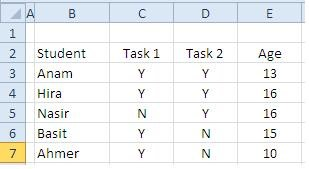# How To Sum Using Logical Functions - An Example Of Using AND () And OR() In Excel### Learn Logical Functions

MS Excel offers several logical functions (also known as logical operators). Using logical functions makes Excel even more versatile. They allow the use of plain language when creating formulas. Today, we will discuss how to use AND () and OR() when summing up values.

### Using the AND function

The AND () function is a logical operator that tests whether multiple conditions are meet. Thus, conceptually, if there are n conditions, all of them should be meet to return a TRUE in the formula. (In contrast, if you are using OR() it implies that either of these could be met to give a TRUE.)

The syntax of the formula is: =AND(logical1,[logical2],…)

The first argument is compulsory and must be provided to evaluate the formula, the second one is optional and one can add up to 256 such conditions. It is to be noted that if any of the conditions are false, the function will return a FALSE. You will only get a TRUE return when all the conditions in the formula are met.### Example: Working out AND()

Let’s consider an example where an examiner wants to know if the student has successfully completed two of the assigned tasks and his age is between 12 and 30 years.

In order to finish the task, the following formula should work:

=IF(AND(C3="Y",D3="Y",AND(E3>=12,E3<=30)),"Passed","Fail")

The inner-most AND(E4>=12,E4<=30) is used to calculate the age bracket. If it falls between 12 and 30, the function will return a TRUE. For the case of Anam, the age is 13. Hence, the formula will evaluate both arguments to be true (greater than or equal to 12 and equal to or less than 30) and return a TRUE.This is passed as a third argument to the outer AND() that checks for the tasks to see if they are completed.

The outer AND() testifies if the tasks are completed by checking for “Y” in columns C and D. We can see that for Anum, both tasks are completed. Hence, there’s a Y in cells C3 and D3. The AND() formula adds these TRUE results to the first TRUE from the age calculation.This triggers the IF() formula and we have result “PASSED” in return.

### Using the OR function

Lets put a twist on the situation. A teacher wants to find the number of students who completed either assignment and is between ages 12 and 30. In this situation, we are compelled to use an OR() condition within the AND() condition. This way, we can specify the student should fall between the ages and have completed either assignment.

### Example: Working out OR()

To calculate this, we can use the following formula:

IF(AND(OR(C3="Y",D3="Y"),AND(E3>=12,E3<=30)),"Passed","Fail")

The only part that has been added is OR(C3="Y",D3="Y") that checks whether either condition is true. When using OR, if either condition returns TRUE, it will result in TRUE. Thus, by altering the previous formula, we can quickly achieve a TRUE result if either task has a Y against it, rather than requiring both to.

Thus concludes this short tutorial on logical functions. If you want to dig deeper into these functions, you can also try out NOR and XOR which execute similar calculations.